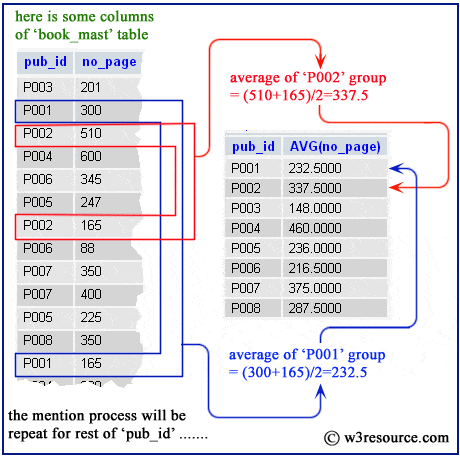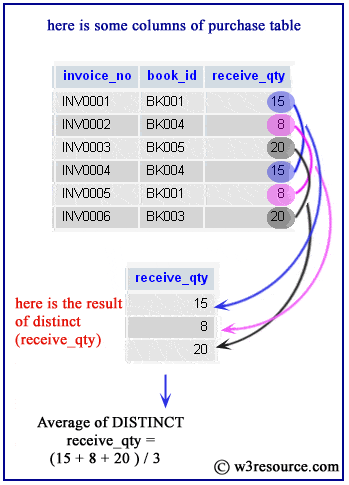# SQLite avg() function

## Description

SQLite avg() function retrieves the average value of a given expression. If the function does not find a matching row, it returns NULL. String and BLOB values that do not look like numbers are interpreted as 0. The result of avg() is always a floating point value as long as at there is at least one non-NULL input even if all inputs are integers. The result of avg() is NULL if and only if there are no non-NULL inputs.

Syntax

`avg([DISTINCT] expr)`

Where expr is a given expression. The DISTINCT option can be used to return the average of the distinct values of expr.

Example: SQLite avg() function

SQLite avg() function with group by and example

SQLite avg() function with distinct and example

SQLite avg() function decimal places and example

SQLite avg() function with count() function and example

SQLite avg() function with having and example

Example: SQLite avg() function

The following SQLite statement will return an average number of pages (of books) from the book_mast table.

Sample table: book_mast

Sample Output:

```sqlite> SELECT avg(no_page) FROM book_mast;
286.625```

Example: SQLite avg() function with group by

SQLite avg() function retrieves the average value of a given expression for each group, if it is used with group by option. The following statement will return the average number of pages for each group of 'pub_id' from book_mast table.

Sample table: book_mast

Sample Output:

```sqlite> SELECT pub_id,avg(no_page) FROM book_mast GROUP BY pub_id;
pub_id      avg(no_page)
----------  ------------
P001        232.5
P002        337.5
P003        148.0
P004        460.0
P005        236.0

P006        216.5
P007        375.0
P008        287.5
```

Pictorial PresentationExample: SQLite avg() function with distinct

SQLite avg() function retrieves the unique average value of a given expression when used with DISTINCT keyword. The following statement will return the average of unique 'receive_qty' from the purchase table.

Sample table: purchase

Sample Output:

```sqlite> SELECT avg(DISTINCT(receive_qty))
...> FROM purchase;
--------------------------
14.3333333333333
```

Pictorial PresentationExample: SQLite avg() function decimal places

Here we have discussed how to use ROUND() along with avg() to retrieve a value calculated upto a specific number of decimal places of a given value. The following statement will return the average number of pages up to 2 decimal places for each group of 'pub_id' from book_mast table.

Sample table: book_mast

Sample Output:

```sqlite> SELECT pub_id,ROUND(avg(no_page),2)
...> FROM book_mast
...> GROUP BY pub_id;
pub_id      ROUND(avg(no_page),2)
----------  ---------------------
P001        232.5
P002        337.5
P003        148.0
P004        460.0
P005        236.0
P006        216.5
P007        375.0
P008        287.5
```

Example: SQLite avg() function with count() function

Here we have discussed how to use SQLite avg() function with count() function to fetch suitable data. The following statement will return the average 'no_page' and number of the publisher for each group of the publisher from book_mast table.

Sample table: book_mast

Sample Output:

```sqlite> SELECT pub_id, avg(no_page)
...> FROM book_mast
...> GROUP BY pub_id;
pub_id      avg(no_page)
----------  ---------------------
P001        232.50
P002        337.50
P003        148.00
P004        460.00
P005        236.00
P006        216.50
P007        375.00
P008        287.50
```

SQLite avg() function with having

SQLite avg() function retrieves the average value of a given expression against a condition specified after HAVING clause for each group specified after the GROUP BY clause. This way you can use HAVING and GROUP BY with SQLite avg() function. The following statement will return the average no_page for those group whose 'pub_id' is 'P008' from the book_mast table.

Sample table: book_mast

Sample Output:

```sqlite> SELECT pub_id, avg(no_page)
...> FROM book_mast
...> GROUP BY pub_id
...> HAVING  pub_id='P008';
pub_id      avg(no_page)
----------  ------------
P008        287.5
```

Previous: Collating Sequences
Next: Count()

﻿